熱身回顧一下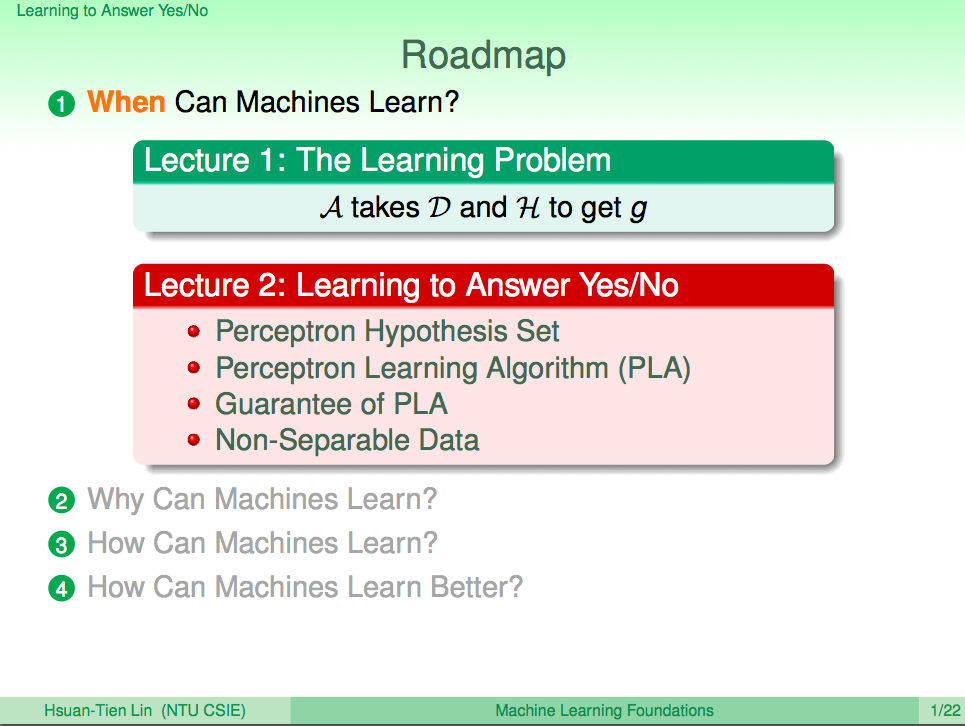再看一次是否要發信用卡這個問題簡單的假設集合：感知器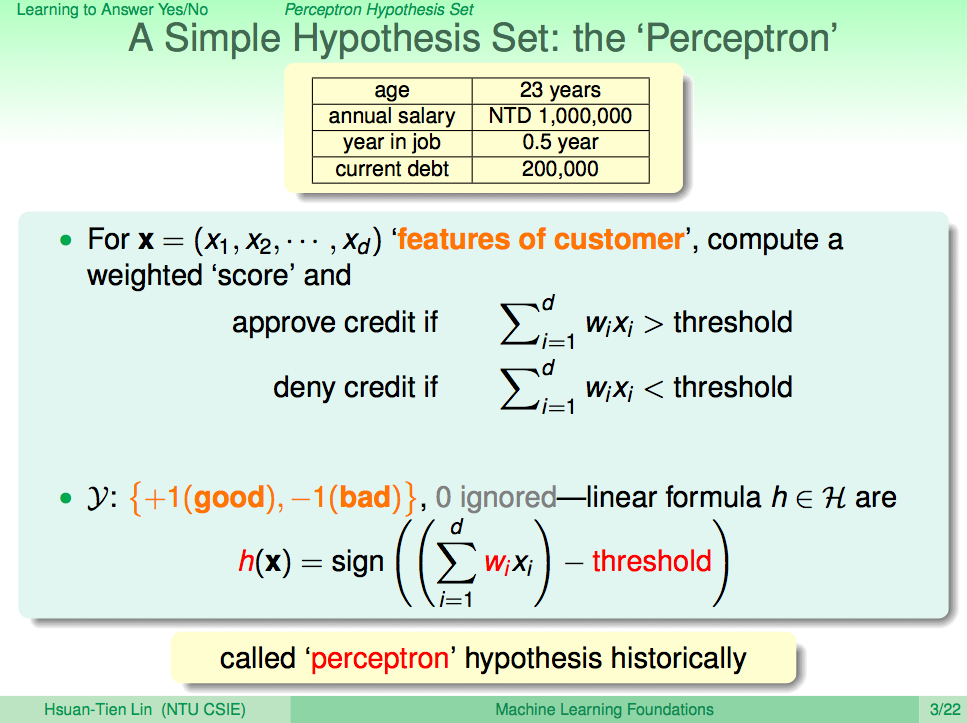將假設集合的線性方程式整理一下

Threshold 我們也可以視為是一種加權分數，所以就可以將假設集合的線性方程式整理得更簡潔，整個線性方程式就變成了很簡易的兩個向量內積而已。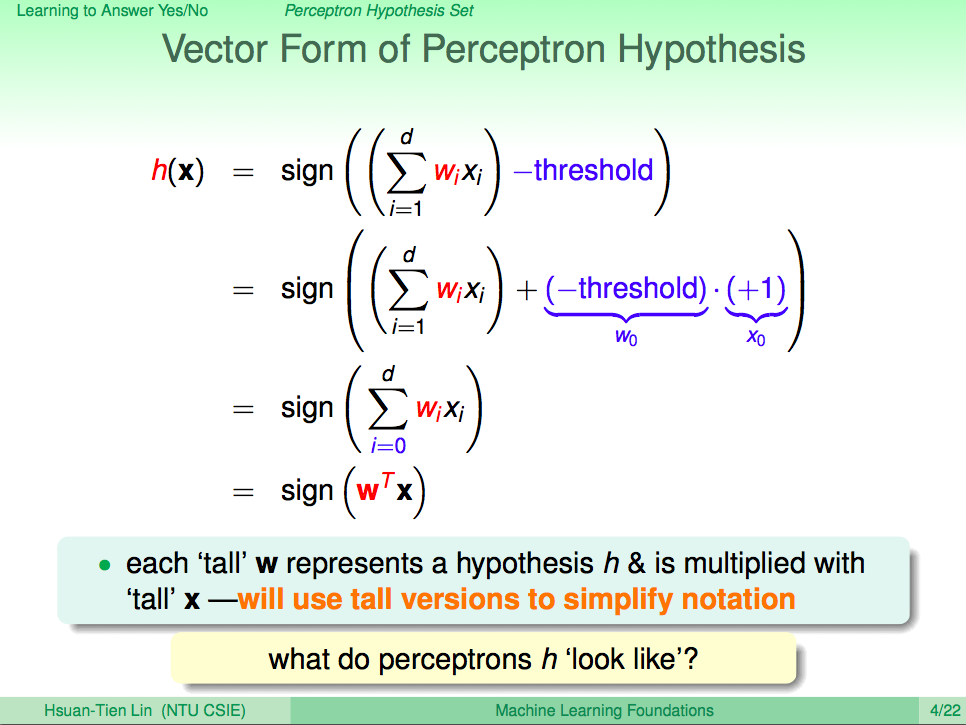用二維空間來看看這個例子怎麼從所有的假設中得到最好的假設 g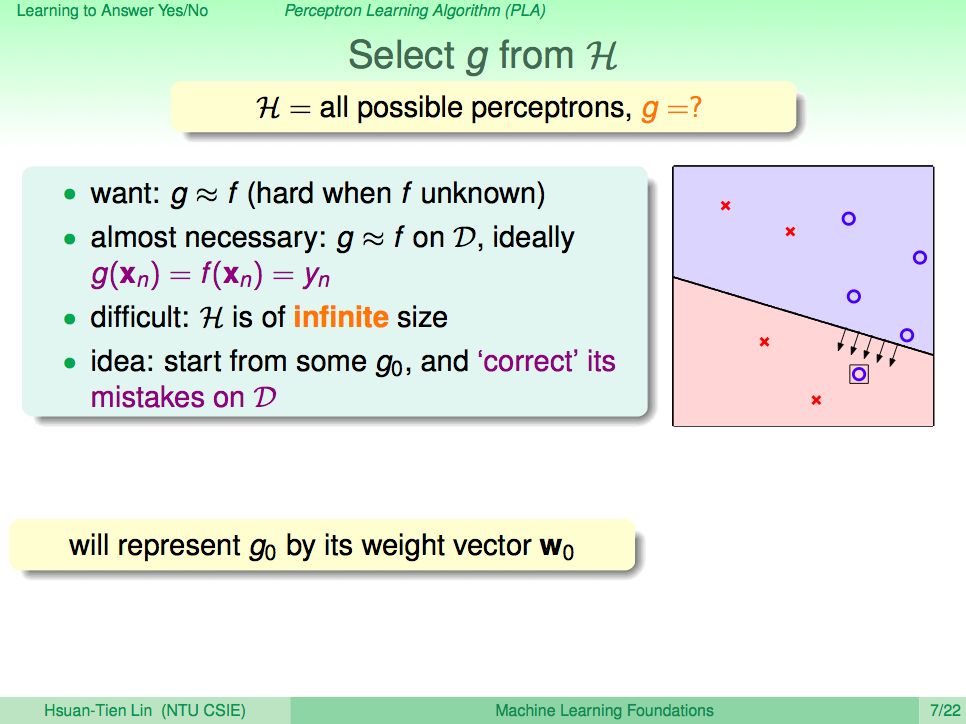Perceptron Learning Algorithm 感知學習模型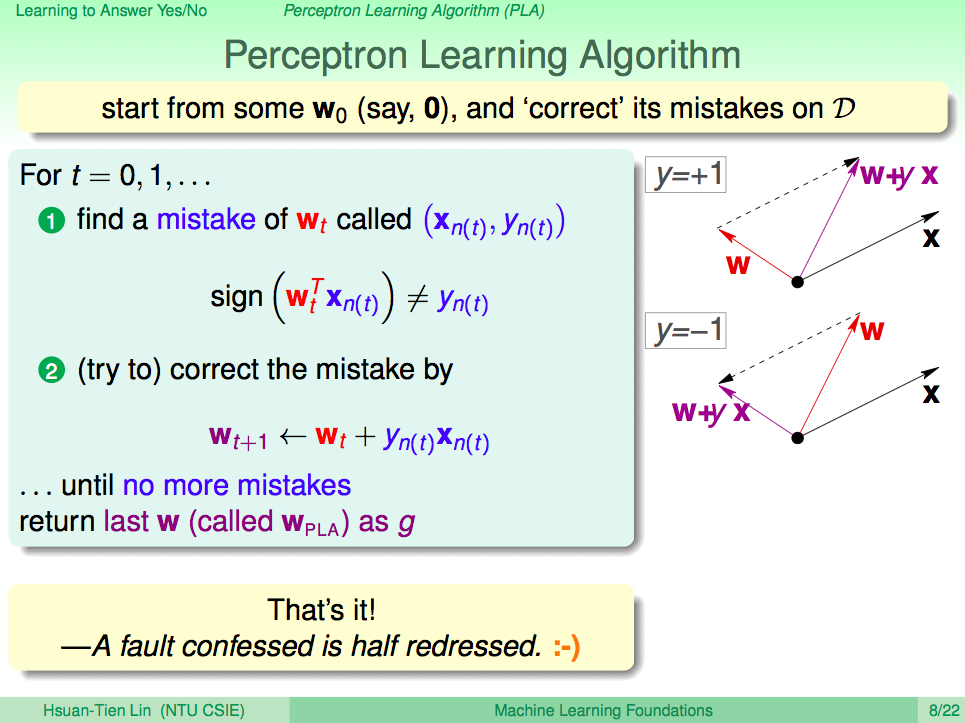看看 PLA 演算法修正 h 的過程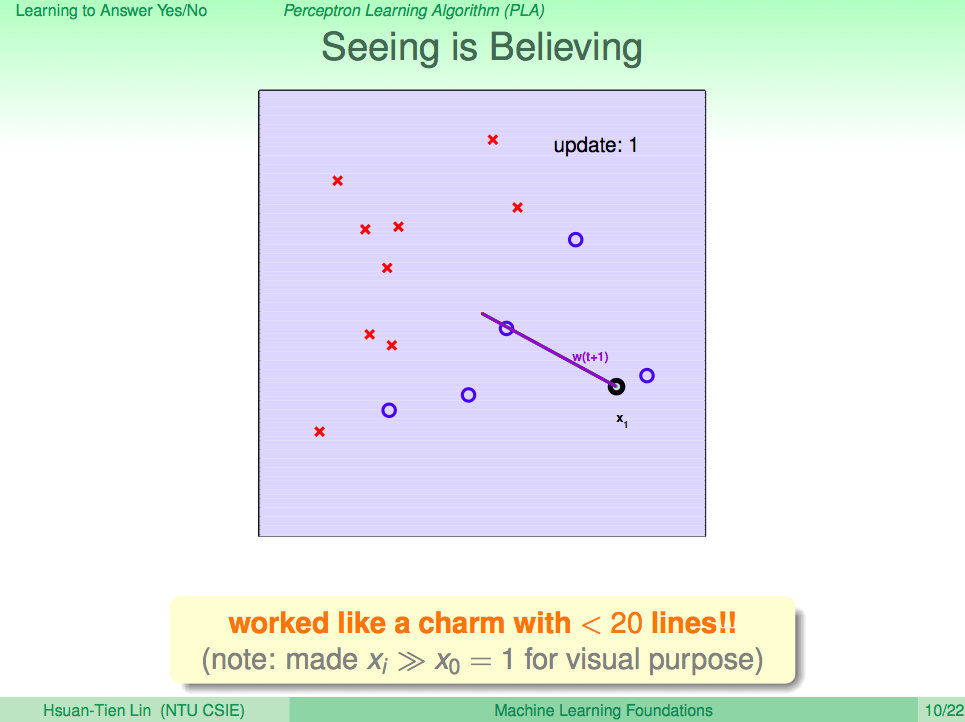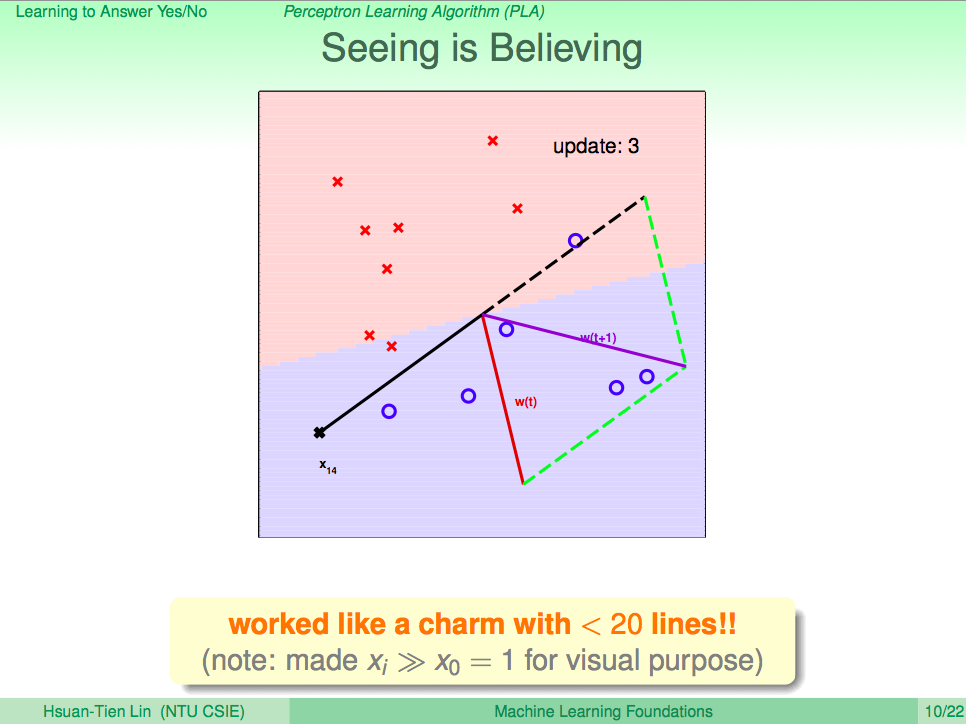PLA 的一些問題

PLA 這個演算法會一直修正 h 直到對所有的 D 都沒有錯誤時，就會停止。但真實世界的資料不會這麼完美，PLA 可能會有不會停止的情況發生，所以我們只能修正 PLA，只算出夠好的 h 就可以了。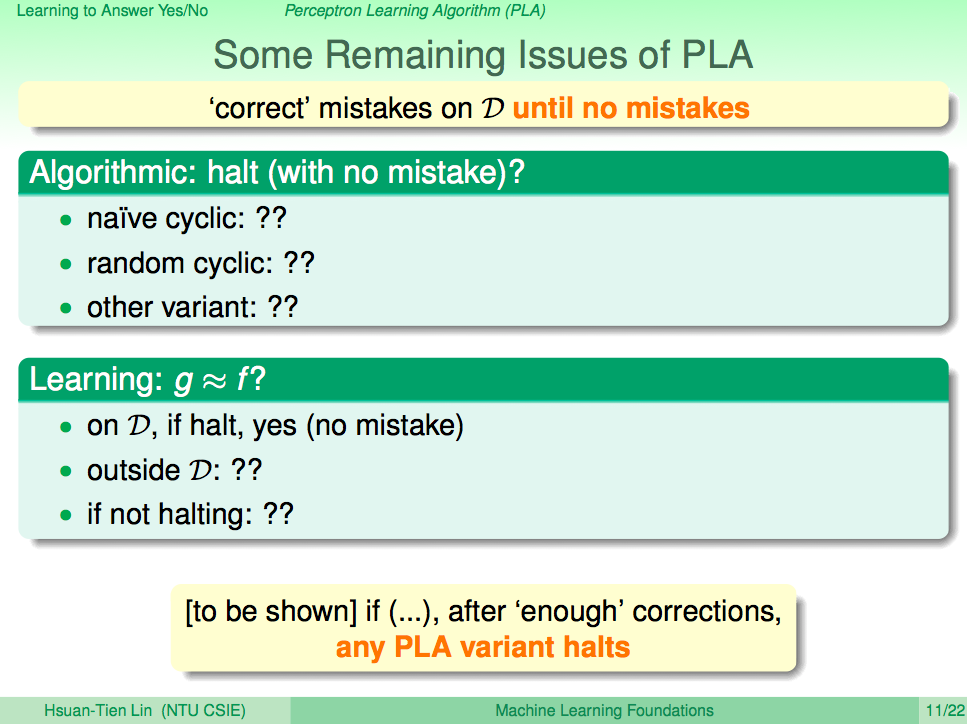什麼時候 PLA 不會停止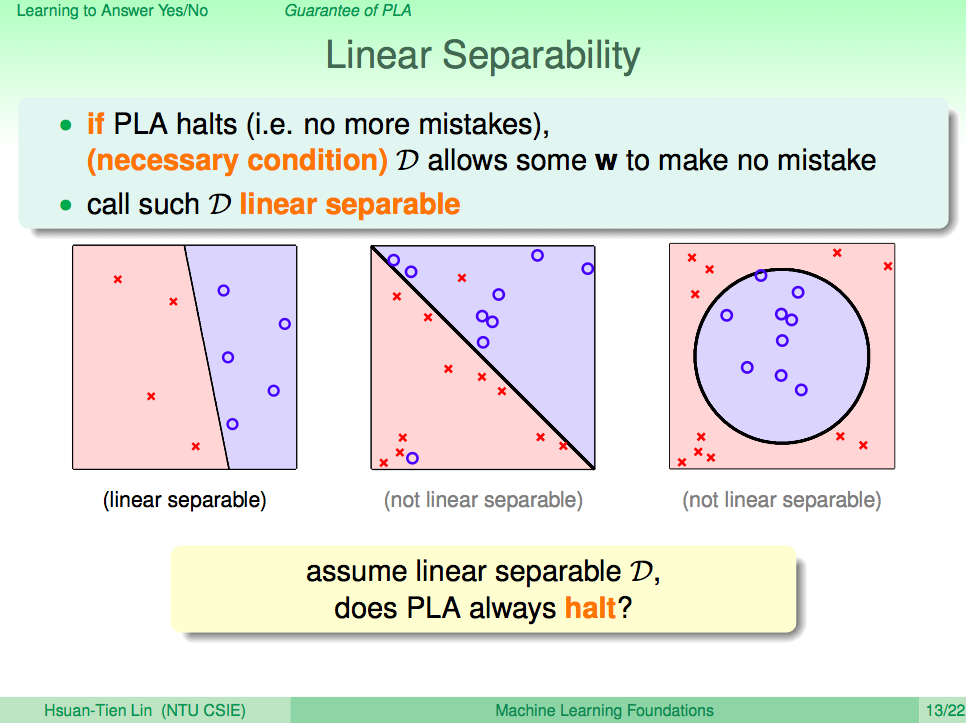處理雜訊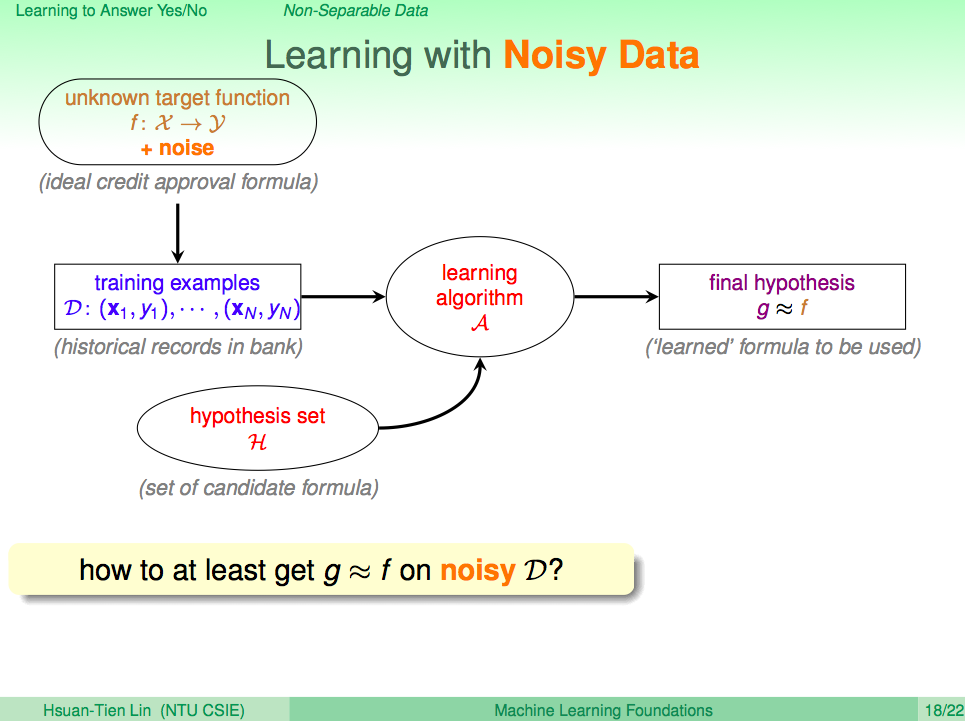找出犯最少錯的線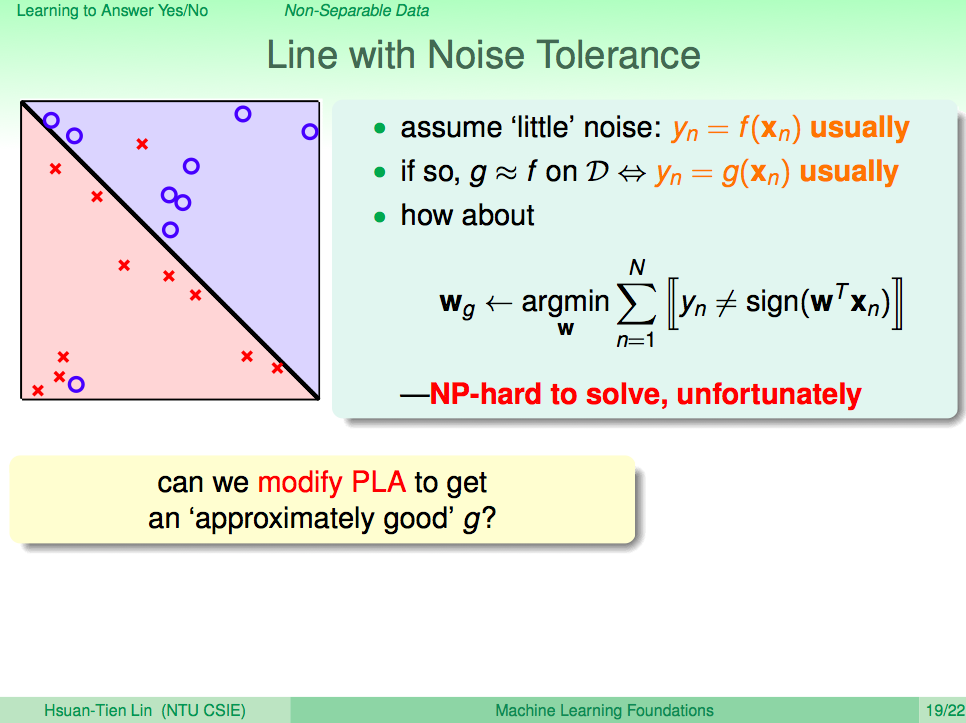Pocket Algorithm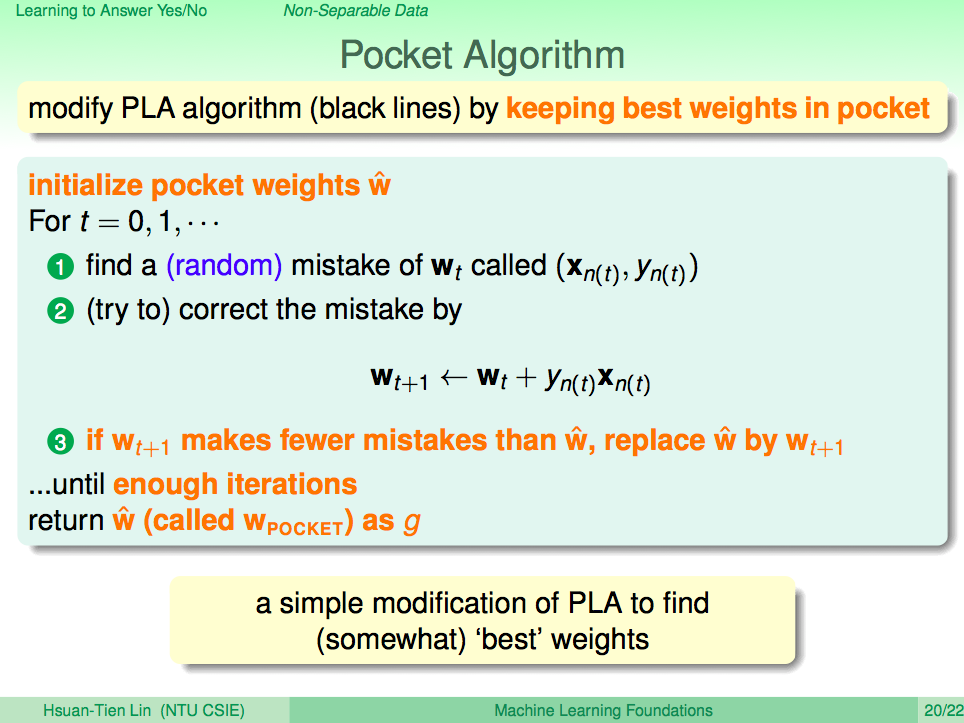演算法原始碼

Naive PLA

``````from numpy import *

def naive_pla(datas):
w = datas
iteration = 0
while True:
iteration += 1
false_data = 0

for data in datas:
t = dot(w, data)
if sign(data) != sign(t):
error = data
false_data += 1
w += error * data
print 'iter%d (%d / %d)' % (iteration, false_data, len(datas))
if not false_data:
break
return w
``````

Pocket PLA

``````import numpy as np

def pocket_pla(datas, limit):
###############
def _calc_false(vec):
res = 0
for data in datas:
t = np.dot(vec, data)
if np.sign(data) != np.sign(t):
res += 1
return res
###############
w = np.random.rand(5)
least_false = _calc_false(w)
res = w

for i in xrange(limit):
data = random.choice(datas)
t = np.dot(w, data)
if np.sign(data) != np.sign(t):
t = w + data * data
t_false = _calc_false(t)

w = t

if t_false <= least_false:
least_false = t_false
res = t
return res, least_false
``````